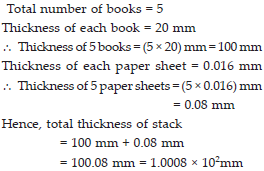# Exponents and Power (Mathematics) Class 8 - NCERT Questions

Q 1.

Evaluate.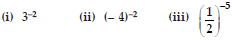SOLUTION: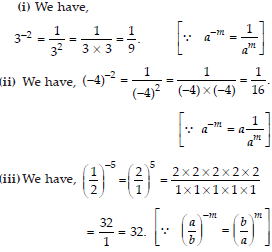Q 2.

Simplify and express the result in power notation with positive exponent.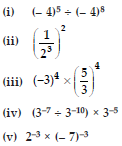SOLUTION: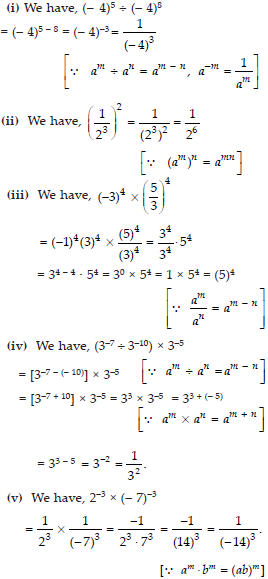Q 3.

Find the value of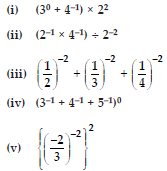SOLUTION: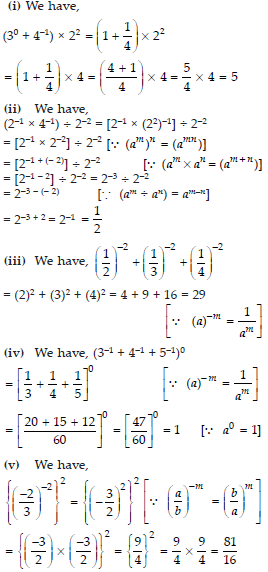Q 4.

Evaluate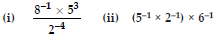SOLUTION: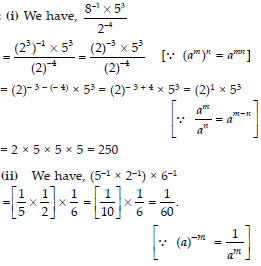Q 5.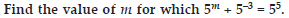SOLUTION: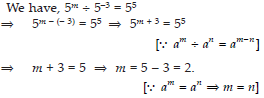Q 6.

Evaluate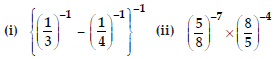SOLUTION: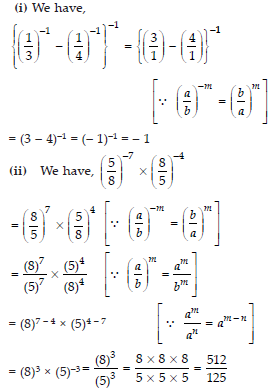Q 7.

SimplifySOLUTION: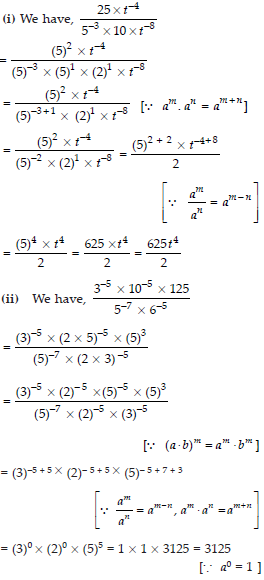Q 8.

Express the following numbers in standard form.
(i) 0.0000000000085
(ii) 0.00000000000942
(iii) 6020000000000000
(iv) 0.00000000837
(v) 31860000000

SOLUTION: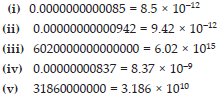Q 9.

Express the following numbers in usual form.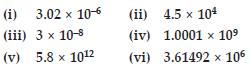SOLUTION: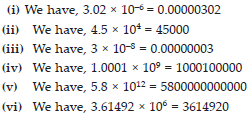Q 10.

Express the number appearing in the following statements in standard form.
(i) 1 micron is equal to 1/1000000m
(ii) Charge of an electron is 0.000,000,000,000,000,000,16 coulomb.
(iii) Size of a bacteria is 0.0000005 m.
(iv) Size of a plant cell is 0.00001275 m.
(v) Thickness of a thick paper is 0.07 mm.

SOLUTION: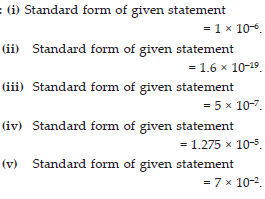Q 11.

In a stack, there are 5 books each of thickness 20 mm and 5 paper sheets each of thickness 0.016 mm. What is the total thickness of the stack.

SOLUTION: